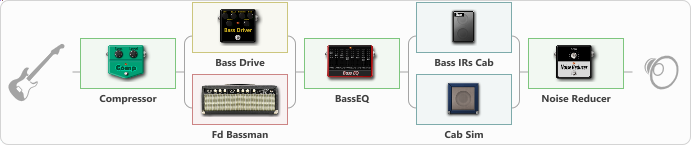# Bass Solo

Discussion in 'ToneLib-GFX presets' started by Peter Bass, Apr 4, 2021.

1. Bass Solo

Preset name: Bass Solo

Effects chain:Effect: "Compressor" (Dynamics / Filter), active - "yes"
{
"Sense" = 21
"Level" = 50
}

Effect: "Splitter" (Dynamics / Filter)
{
"A-Bypass" = Off
"A-Pan" = -14
"A-Level" = 55
"B-Bypass" = Off
"B-Pan" = 14
"B-Level" = 55

'A' branch:
{

Effect: "Bass Drive" (Overdrive / Distortion), active - "yes"
{
"Drive" = 100
"Blend" = 100
"Presence" = 10
"Bass" = 74
"Middle" = 26
"Treble" = 50
"Level" = 78
}
}
'B' branch:
{

Effect: "Fd Bassman" (Amp simulators), active - "yes"
{
"Gain" = 34
"Bass" = 86
"Middle" = 18
"Treble" = 50
"Presence" = 58
"Master" = 50
"Level (dB)" = 4
}
}
}

Effect: "BassEQ" (Dynamics / Filter), active - "yes"
{
"50 Hz" = 9
"120 Hz" = 3
"400 Hz" = 5
"500 Hz" = -5
"800 Hz" = -5
"4.5 kHz" = -5
"10 kHz" = 0
"Level (dB)" = 9
}

Effect: "Splitter" (Dynamics / Filter)
{
"A-Bypass" = Off
"A-Pan" = -35
"A-Level" = 55
"B-Bypass" = Off
"B-Pan" = 35
"B-Level" = 55

'A' branch:
{

Effect: "Bass IRs Cab" (Cabinets), active - "yes"
{
"Model" = Markbass combo (1x12")
"Mic Position" = Edge
"Mic Distance" = Middle
"Low Cut (Hz)" = 54
"Hi Cut (kHz)" = 9.5
"Mix" = 100
"Level (dB)" = 0
}
}
'B' branch:
{

Effect: "Cab Sim" (Cabinets), active - "yes"
{
"Model" = 4x10" Tweed P10r
"Level (dB)" = 0
}
}
}

Effect: "Noise Reducer" (Dynamics / Filter), active - "yes"
{
"Sens" = 74
"Mode" = Hard
}

Note: You will need to download and install the ToneLib-GFX software to use the preset.

#### Attached Files:

• ###### Bass_Solo.tlgfx
File size:
893 bytes
Views:
2,663
armaan likes this.